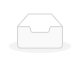News

17

2020

-

11

# Conditioning valve flow characteristics of the selection method

Tanggu valve is mainly divided into electric Tanggu valve and pneumatic Tanggu valve two categories. Tanggu valve flow characteristics, refers to the pressure difference between the two ends of the valve to maintain stable conditioning, the relative flow of the medium through the Tanggu valve and its opening.

Tanggu valve flow characteristics have linear characteristics, the same percentage characteristics and parabolic characteristics of three. The meanings of the three injection volume characteristics are as follows:

Equal percentage characteristics (logarithm) : equal percentage characteristics of the relative stroke and relative flow is not linear connection, at each point in the stroke of the unit stroke change caused by the change in the flow rate is proportional to the flow of this point, the change in the flow ratio is equal. So its advantage is that when the flow is small, the flow is small, and when the flow is large, the flow is large, that is, it has the same conditioning accuracy in different opening.

2, linear characteristics (linear) : linear characteristics of the relative stroke and relative flow in a straight line. The change in flow rate per unit trip is constant. When the flow is large, the relative value of the flow is small, and when the flow is small, the relative value of the flow is large.

3, parabolic characteristics: flow according to the stroke of the square into the share of change, roughly has the central characteristics of linear and equal percentage characteristics.

From the analysis of the above three characteristics, it can be seen that in terms of its conditioning performance, the equal percentage characteristic is the best, its conditioning stability, conditioning performance is good. The parabolic characteristic is better than the linear characteristic of conditioning performance, according to the requirements of the application of different occasions, choose any of the flow characteristics.undefined